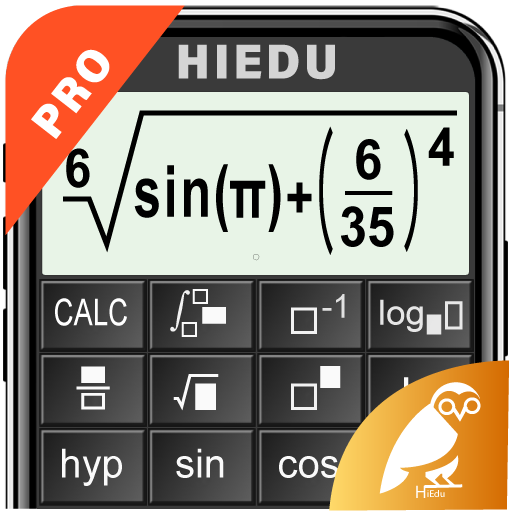# HiEdu Scientific Calculator Pro MOD v1.2.3 (Paid Full Version)

Genres
Version
1.2.3
Requires
Android 4.1 and up
Size
5.4M
MOD Features
Paid Full Version
Updated
September 16, 2021
Facing Issue? Report

# HiEdu Scientific Calculator Pro MOD v1.2.3 (Paid Full Version)

### Download The Latest Apk Version Of HiEdu Scientific Calculator Pro MOD v1.2.3 (Paid Full Version), A none App For Your Android Device. This Mod Includes Paid Full Version Features Unlocked.

HiEdu Scientific Calculator Pro : A simple yet powerful calculator that includes standard, scientific, and programmer modes, as well as a unit converter, Math, Physics and Chemistry Formulas, graph and solve the equations . It’s the perfect tool to add up a bill, convert measurements in a recipe or other project and It is a very useful tool for education help complete complex math, algebra, or geometry problems.

KEY FEATURES
Scientific Calculator
• It is a scientific calculator supports most of the features of real calculators.
• Natural Display makes it possible to input and display fractions and certain functions (log, x2, x3, x^, ), “, x−1, 10^, e^ , d/dx, Σ, Abs …) just as they are written in your textbook.
• Undo when you miss a mistake.
• Save history, Select a calculation in list history and edit it.
• Create favorite calculations that make calculations faster.

Standard Calculator
• This is a perfect tool for daily calculations. With memory functions similar to a small handheld calculator
• Save history, Select a calculation in list history and edit it.

Mathematics formulas
• This app has 1000+ math formula and more to come.
• Now no need to make paper notes to remember mathematics formulas just have this app put all the formulas on your favourite phones.
• you’ll find formulas very simply explained in app with necessary figures will help you to understand very easily.

Physics formulas
• The application is a physical handbook. Contains most physical formulas for students and undergraduate.help users quickly refer to any Physics formulas for their study and work.This app displays most popular as well as advanced formulas in seven categories:
+ Mechanics
+ Electricity
+ Thermal physics
+ Periodic motion
+ Optics
+ Atomic physics
+ Constants

Chemical reactions
• Allows to discover chemical reactions and to solve the chemical equations with one and several unknown variables.

Programmers
• Can convert numbers between different number bases (2/8/10/16).
• Display shows numbers in binary, hexadecimal, octal and decimal.
• Input can be in binary, hexadecimal, octal or decimal.

Graphing calculator
• Easily graph functions, solve equations, find special points of functions.

Solve the equations
• Linear equation for degree one,quadratic equation for degree two,cubic equation for degree three, System of linear equations.

Converter
• Available unit conversions include:
+ Currency (US dollar, CDN dollar, pound, peso, etc)
+ Temperature (celsius, fahrenheit, kelvin, etc)
+ Length (kilometer, miles, meter, yard, feet, etc)
+ Mass/Weight (kilogram, pound, ounce, ton, stone, etc)
+ Speed (km/h, mph, knot, etc)
+ Area (square kilometer, square mile, hectare, acre, etc)
+ Cooking Volume (teaspoon, tablespoon, cup, pint, quart, ounce, etc)
+ Pressure (kilopascal, bar, PSI, etc)
+ Power (watt, kilowatt, horsepower, etc)
+ Energy (joule, calorie, BTU, etc)
+ Time (year, month, day, hour, second, etc)
+ Fuel Consumption (miles per gallon, liters per 100km, etc)
+ Digital Storage (bit, byte, megabytes, gigabytes, etc)

DISPLAY
+ The layout of the buttons in the this app is scientifically rigorous, which makes entering mathematical operations as simple and convenient as possible.
+ Numeric buttons and functions in the our application are beautifully designed, clear, resistant to glare, blurry and eyestrain.
+ There are many beautiful themes with different styles.

## How to Install & Download HiEdu Scientific Calculator Pro MOD Apk

Step 3 Enable Unknown Source: Till your downloading in process, Enable the Unknown source settings of your android phone so that you can install this third party application.